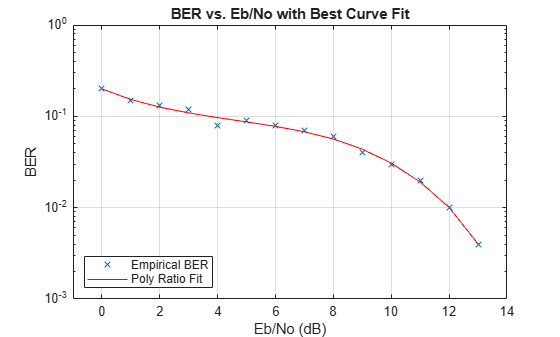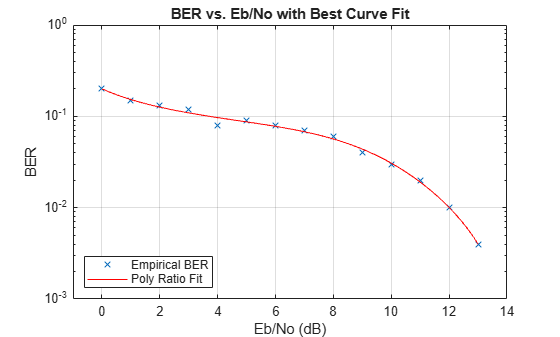Documentation

# berfit

Fit curve to nonsmooth empirical bit error rate (BER) data

## Syntax

```fitber = berfit(empEbNo,empber) fitber = berfit(empEbNo,empber,fitEbNo) fitber = berfit(empEbNo,empber,fitEbNo,options) fitber = berfit(empEbNo,empber,fitEbNo,options,fittype) [fitber,fitprops] = berfit(...) berfit(...) berfit(empEbNo,empber,fitEbNo,options,'all') ```

## Description

`fitber = berfit(empEbNo,empber)` fits a curve to the empirical BER data in the vector `empber` and returns a vector of fitted bit error rate (BER) points. The values in `empber` and `fitber` correspond to the Eb/N0 values, in dB, given by `empEbNo`. The vector `empEbNo` must be in ascending order and must have at least four elements.

### Note

The `berfit` function is intended for curve fitting or interpolation, not extrapolation. Extrapolating BER data beyond an order of magnitude below the smallest empirical BER value is inherently unreliable.

`fitber = berfit(empEbNo,empber,fitEbNo)` fits a curve to the empirical BER data in the vector `empber` corresponding to the Eb/N0 values, in dB, given by `empEbNo`. The function then evaluates the curve at the Eb/N0 values, in dB, given by `fitEbNo` and returns the fitted BER points. The length of `fitEbNo` must equal or exceed that of `empEbNo`.

`fitber = berfit(empEbNo,empber,fitEbNo,options)` uses the structure `options` to override the default options used for optimization. These options are the ones used by the `fminsearch` function. You can create the `options` structure using the `optimset` function. Particularly relevant fields are described in the table below.

FieldDescription
`options.Display`Level of display: `'off'` (default) displays no output; `'iter'` displays output at each iteration; `'final'` displays only the final output; `'notify'` displays output only if the function does not converge.
`options.MaxFunEvals`Maximum number of function evaluations before optimization ceases. The default is 104.
`options.MaxIter`Maximum number of iterations before optimization ceases. The default is 104.
`options.TolFun`Termination tolerance on the closed-form function used to generate the fit. The default is 10-4.
`options.TolX`Termination tolerance on the coefficient values of the closed-form function used to generate the fit. The default is 10-4.

`fitber = berfit(empEbNo,empber,fitEbNo,options,fittype)` specifies which closed-form function `berfit` uses to fit the empirical data, from the possible fits listed in Algorithms below. `fittype` can be `'exp'`, `'exp+const'`, `'polyRatio'`, or `'doubleExp+const'`. To avoid overriding default optimization options, use `options = []`.

`[fitber,fitprops] = berfit(...)` returns the MATLAB structure `fitprops`, which describes the results of the curve fit. Its fields are described in the table below.

FieldDescription
`fitprops.fitType`The closed-form function type used to generate the fit: `'exp'`, `'exp+const'`, `'polyRatio'`, or `'doubleExp+const'`.
`fitprops.coeffs`The coefficients used to generate the fit. If the function cannot find a valid fit, `fitprops.coeffs` is an empty vector.
`fitprops.sumSqErr`The sum squared error between the log of the fitted BER points and the log of the empirical BER points.
`fitprops.exitState`The exit condition of `berfit`: ```'The curve fit converged to a solution.'```, ```'The maximum number of function evaluations was exceeded.'```, or ```'No desirable fit was found'```.
`fitprops.funcCount`The number of function evaluations used in minimizing the sum squared error function.
`fitprops.iterations`The number of iterations taken in minimizing the sum squared error function. This is not necessarily equal to the number of function evaluations.

`berfit(...)` plots the empirical and fitted BER data.

`berfit(empEbNo,empber,fitEbNo,options,'all')` plots the empirical and fitted BER data from all the possible fits, listed in the Algorithms below, that return a valid fit. To avoid overriding default options, use `options = []`.

### Note

A valid fit must be

• real-valued

• monotonically decreasing

• greater than or equal to 0 and less than or equal to 1

If a fit does not confirm to this criteria, it is rejected.

## Examples

collapse all

These examples illustrate the syntax of the `berfit` function, but they use hard-coded or theoretical BER data for simplicity. For an example that uses empirical BER data from a simulation, see Curve Fitting An Error Rate Plot.

Best fit for a sample set of data

```EbN0 = 0:13; berdata = [.2 .15 .13 .12 .08 .09 .08 .07 .06 .04 .03 .02 .01 .004]; berfit(EbN0,berdata); ```Plot the best fit. The curve connects the points created by evaluating the fit expression at the values in EbN0. To make the curve look smoother, use a syntax like `berfit(EbN0,berdata,[0:0.2:13])`. This alternative syntax uses more points when plotting the curve, but it does not change the fit expression.

Fit for a BER curve with an error floor

We generate the empirical BER array by simulating a channel with a null (ch = [0.5 0.47]) with BPSK modulation and linear MMSE equalizer at the receiver. We run the berfit with the 'all' option. The `'doubleExp+const'` fit does not provide a valid fit, and the `'exp'` fit type does not work well for this data. The `'exp+const'` and `'polyRatio'` fits closely match the simulated data.

```EbN0 = -10:3:15; empBER = [0.3361 0.3076 0.2470 0.1878 0.1212 0.0845 0.0650 0.0540 0.0474]; figure; berfit(EbN0, empBER, [], [], 'all');```Use of the `options` input structure as well as the `fitprops` output structure

The `'notify'` value for the display level causes the function to produce output when one of the attempted fits does not converge. The `exitState` field of the output structure also indicates which fit converges and which fit does not.

```M = 8; EbN0 = 3:10; berdata = berfading(EbN0,'psk',M,2); % Compute theoretical BER. noisydata = berdata.*[.93 .92 1 .59 .08 .15 .01 .01]; % Say when fit fails to converge. options = optimset('display','notify'); disp('*** Trying exponential fit.') % Poor fit```
```*** Trying exponential fit. ```
```[fitber1,fitprops1] = berfit(EbN0,noisydata,EbN0,... options,'exp')```
``` Exiting: Maximum number of function evaluations has been exceeded - increase MaxFunEvals option. Current function value: 2.749919 ```
```fitber1 = 1×8 0.1247 0.0727 0.0376 0.0168 0.0064 0.0020 0.0005 0.0001 ```
```fitprops1 = struct with fields: fitType: 'exp' coeffs: [4x1 double] sumSqErr: 2.7499 exitState: 'The maximum number of function evaluations has been exceeded' funcCount: 10001 iterations: 6193 ```
`disp('*** Trying polynomial ratio fit.') % Good fit`
```*** Trying polynomial ratio fit. ```
```[fitber2,fitprops2] = berfit(EbN0,noisydata,EbN0,... options,'polyRatio')```
```fitber2 = 1×8 0.1701 0.0874 0.0407 0.0169 0.0060 0.0016 0.0003 0.0001 ```
```fitprops2 = struct with fields: fitType: 'polyRatio' coeffs: [6x1 double] sumSqErr: 2.3880 exitState: 'The curve fit converged to a solution' funcCount: 554 iterations: 331 ```

## Algorithms

The `berfit` function fits the BER data using unconstrained nonlinear optimization via the `fminsearch` function. The closed-form functions that `berfit` considers are listed in the table below, where x is the Eb/N0 in linear terms (not dB) and f is the estimated BER. These functions were empirically found to provide close fits in a wide variety of situations, including exponentially decaying BERs, linearly varying BERs, and BER curves with error rate floors.

Value of `fittype`Functional Expression
`'exp'`$f\left(x\right)={a}_{1}\mathrm{exp}\left[\frac{-{\left(x-{a}_{2}\right)}^{{a}_{3}}}{{a}_{4}}\right]$
`'exp+const'`$f\left(x\right)={a}_{1}\mathrm{exp}\left[\frac{-{\left(x-{a}_{2}\right)}^{{a}_{3}}}{{a}_{4}}\right]+{a}_{5}$
`'polyRatio'`

`$f\left(x\right)=\frac{{a}_{1}{x}^{2}+{a}_{2}x+{a}_{3}}{{x}^{3}+{a}_{4}{x}^{2}+{a}_{5}x+{a}_{6}}$`

`'doubleExp+const'`$\begin{array}{l}{a}_{1}\mathrm{exp}\left[\frac{-{\left(x-{a}_{2}\right)}^{{a}_{3}}}{{a}_{4}}\right]\\ \begin{array}{cc}& \end{array}+{a}_{5}\mathrm{exp}\left[\frac{-{\left(x-{a}_{6}\right)}^{{a}_{7}}}{{a}_{8}}\right]+{a}_{9}\end{array}$

The sum squared error function that `fminsearch` attempts to minimize is

where the fitted BER points are the values in `fitber` and the sum is over the Eb/N0 points given in `empEbNo`. It is important to use the log of the BER values rather than the BER values themselves so that the high-BER regions do not dominate the objective function inappropriately.

## References

For a general description of unconstrained nonlinear optimization, see the following work.

 Chapra, Steven C., and Raymond P. Canale, Numerical Methods for Engineers, Fourth Edition, New York, McGraw-Hill, 2002.